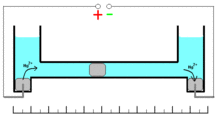# Mercury coulometer

﻿
Mercury coulometer

Mercury coulometer is an electroanalytical chemistry device using mercury to determine the amount of matter transformed (in coulombs) during the following reaction:

Hg2+ + 2e = Hgo

These oxidation/reduction processes have 100% efficiency with the wide range of the current densities. Measuring of the quantity of electricity (coulombs) is based on the changes of the mass of the mercury electrode. Mass of the electrode can be increased during cathodic deposition of the mercury ions or decreased during the anodic dissolution of the metal.$Q = \frac{ \Delta\ m*2*F}{M}$,

where

Q-quantity of electricity (coulombs);$\Delta\ m$-mass changes (gr);
F-Faraday constant (96485 Coulomb.Mol– 1) and
M the molar mass of mercury: (200.59 gr.Mol– 1);

## ConstructionThis coulometer has different constructions but all of them are based on mass measurements. One of the most significant construction is shown on the picture. It consists of two reservoirs connected by a thin graduated capillary. All system contains solution of the mercury(II)-ions solution. Each of the reservoirs have an electrode immersed into a drop of mercury. Another small drop of mercury is inserted into the capillary. When the current is turned on, it will initiate dissolution of the metallic mercury on the one side of the drop in the capillary and deposition on the other side of the same drop. This drop starts to move. Because of the 100% efficiency of the deposition/dissolution of the mercury under the current influence, mass or volume of this small drop will be a constant and its movement will be linearly correlated with the passed charge. If you change direction of the current, the drop starts move in opposite direction. Sensitivity of this type of coulometers depends on the diameter of the capillary.

1. ^ Although the indication of the zero charge of the metal is not needed, in discussing situations with charged AND uncharged particles, it clearly indicates the charge is not "forgotten".

Wikimedia Foundation. 2010.

### Look at other dictionaries:

• Mercury switch — A Single Pole, Single Throw (SPST) mercury switch on millimetre graph paper …   Wikipedia

• Copper coulometer — The copper coulometer is a one of the common application of the copper copper(II) sulfate electrode. Such a coulometer consists of two identical copper electrodes immersed in slightly acidic pH buffered solution of copper(II) sulfate. Passing of… …   Wikipedia

• Coulometry — is the name given to a group of techniques in analytical chemistry that determine the amount of matter transformed during an electrolysis reaction by measuring the amount of electricity (in coulombs) consumed or produced. There are two basic… …   Wikipedia

• Cyclic voltammetry — Typical cyclic voltammogram where ipc and ipa show the peak cathodic and anodic current respectively for a reversible reaction. Cyclic voltammetry or CV is a type of potentiodynamic electrochemical measurement. In a cyclic voltammetry experiment… …   Wikipedia

• Differential pulse voltammetry — (AKA Differential Pulse Polarography or DPP) is often used to make electrochemical measurements. It can be considered as a derivative of linear sweep voltammetry or staircase voltammetry, with a series of regular voltage pulses superimposed on… …   Wikipedia

• Potentiometer (measuring instrument) — A potentiometer is an instrument for measuring the potential (voltage) in a circuit. Before the introduction of the moving coil and digital volt meters, potentiometers were used in measuring voltage, hence the meter part of their name. The method …   Wikipedia